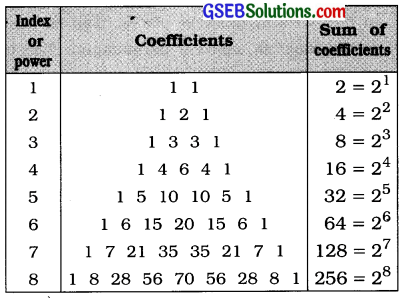# GSEB Class 11 Statistics Notes Chapter 6 Permutations, Combinations and Binomial Expansion

This GSEB Class 11 Commerce Statistics Notes Chapter 6 Permutations, Combinations and Binomial Expansion covers all the important topics and concepts as mentioned in the chapter.

## Permutations, Combinations and Binomial Expansion Class 11 GSEB Notes

Basic Principle of Counting:

• Basic Principle of Counting for Addition: If there are m distinct things in Group 1 and n distinct things in Group 2, then selection of one thing from total things of both groups can be done in m + n ways.
• Basic Principle of Counting for Multiplication: If the first operation can be done in m ways and corresponding to it second operation can be done in n ways, then two operations together can be done in m × n ways.
• General form of basic principle: If the first operation can be done in m ways, following which the second operation can be done in n ways and following which the third operation can be done in p ways then the total number of ways of occurrence of three operation together is m × n × p.

Meaning of Permutation:
If r distinct things out of given n distinct things are to arranged in r (1 ≤ r ≤ n) different places, then each such arrangement is called a permutation. The total number of such arrangements is denoted by npr .npr p(n, r)

• Order of the things is very important in permutation. AB and BA are two different permutations.
• nPr = n(n – 1) (n – 2) … (n – r + 1); 1 ≤ r ≤ n

Factorial:
The multiplication of numbers 1 to n, in their respective order, is called n factorial. It is denoted by n!.

• n! = n × (n – 1) × (n – 2)×… ×3 × 2 × 1
• 0! = 1 and 1! = 1

In factorial form: nPr = $$\frac{n !}{(n-r) !}$$; n > 0, r ≥ 0, n ≥ r. n and r positive integers.

Some Results:
nP0 = 1, nPn = n !, nP1 = n, nPn-1 = n!

Permutations of identical things:
Prom n things, if p is the first type of identical things, q is the second type of identical things and r is the third type of indentical things then arrangement of n things are called permutations of identical objects.
Here, total number of permutation for n things = $$\frac{n !}{p ! q ! r !}$$Meaning of Combination:
The total number of selection of r (r ≤ n) things out of n different things is called combination. It is denoted by nCr

• Here order is ignored. AB and BA are considered as same selection.
• nCr = $$\frac{n !}{r !(n-r) !}$$
• nC0 = 1; nCn = 1; nCr = nCn-r
nC1 = n, nCn-1 = n
• If nCx = nCy, then x + y = n OR x = y.

Meaning of Binomial Expansion:

• Binomial Expression: Any expression consisting of two terms connected by + or – sign is called binomial expression.
• Binomial Expansion: The expansion of binomial expression (x + a) with positive integer power n is called binomial expansion, which is as follows:
• (x + a)n = nC0xn + nC1xn-1a + nC2xn-2 a2 + nC3 xn-3 a3 + … + nCnan
• General terms of binomial expansion is Tr+1 = nCr xn-r. ar

Characteristic of Binomial Expansion:

• The total number of terms in binomial expansion is (n + 1).
• In successive terms of expansion, the power of x keeps reducing by one while power of a keeps increasing by one.
• In any term of expansion, the sum of power of x and a is n.
• The coefficients of successive terms of expansion are nC0, nC1, nC2…….. nCn.
• The coefficients of terms, placed equidistant from the midterm, are equal.

Important Results
1. Permutations:

• nPr = n(n- 1) (n-2)… (n-r + 1)
• nPr = $$\frac{n !}{(n-r) !}$$
• nPn = n !
• n ! = n(n- 1) (n-2) (n-3) … × 3 × 2 × 1
• Identical permutations :
Total permutations of ‘n’ things = $$\frac{n !}{p ! q ! r !}$$

2. Combination:

• nCr = $$\frac{n !}{r !(n-r) !}$$
• nC0 = 1, nC1 = n, nCn-1 = n
• nCn = 1
• nCr = nCn-r
• If nCx = nCy. then x + y = n or x = y
• nCr + nCr-1 = n+1Cr
• nC0 + nC1 + … + nCn = 2n

3. Binomial expansion:

• (x + a)n = nC0xn + nC1xn-1a + nC2xn-2a2 + nC3xn-3a3 + … + nCnan
• (x – a)n = nC0xnnC1xn-1a + nC2xn-2a2nC3xn-3a3 + … ± nCnan4. General term of Binomial expansion:
General term = nCr xn-r ar.
This term is (r + 1)th term of binomial expansion.

5. Some results:

• If n is even:
The middle term of the expansion (x + a)n is obtained by putting r = $$\frac{n}{2}$$ in the general term.
• If n is odd:
Two middle terms of the expansion of (x + a)n is nC0 + nC1 + nC2 + ……… + nCn = 2n

6. Paseals triangle for coefficients of Binomial expansion: